# monitoring

## 2022年10月

Posted by franztao on October 27, 2022

## 系统运行状况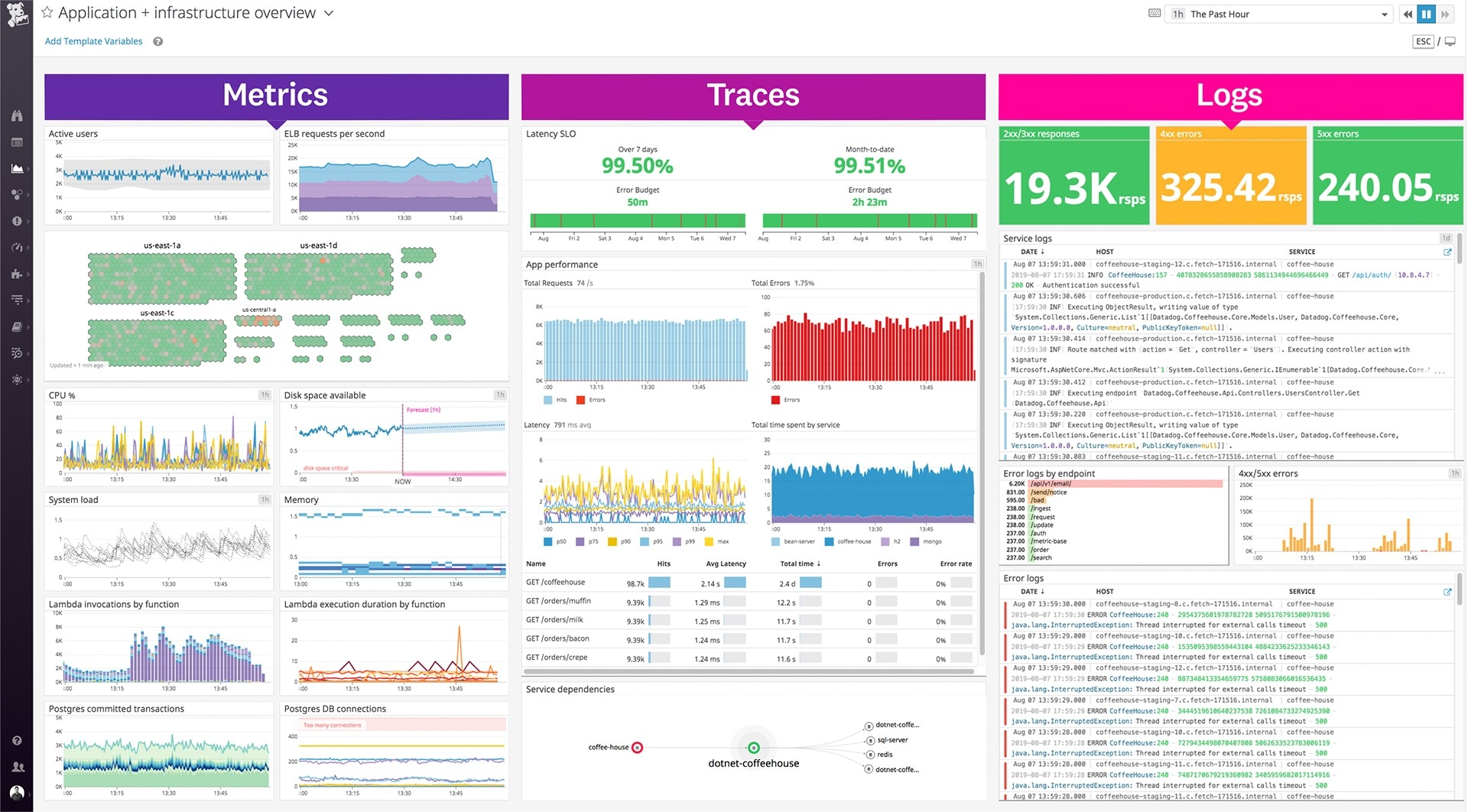## 表现

import matplotlib.pyplot as plt
import numpy as np
import seaborn as sns
sns.set_theme()

# Generate data
hourly_f1 = list(np.random.randint(low=94, high=98, size=24*20)) + \
list(np.random.randint(low=92, high=96, size=24*5)) + \
list(np.random.randint(low=88, high=96, size=24*5)) + \
list(np.random.randint(low=86, high=92, size=24*5))

# Cumulative f1
cumulative_f1 = [np.mean(hourly_f1[:n]) for n in range(1, len(hourly_f1)+1)]
print (f"Average cumulative f1 on the last day: {np.mean(cumulative_f1[-24:]):.1f}")

# Sliding f1
window_size = 24
sliding_f1 = np.convolve(hourly_f1, np.ones(window_size)/window_size, mode="valid")
print (f"Average sliding f1 on the last day: {np.mean(sliding_f1[-24:]):.1f}")

plt.ylim([80, 100])
plt.hlines(y=90, xmin=0, xmax=len(hourly_f1), colors="blue", linestyles="dashed", label="threshold")
plt.plot(cumulative_f1, label="cumulative")
plt.plot(sliding_f1, label="sliding")
plt.legend()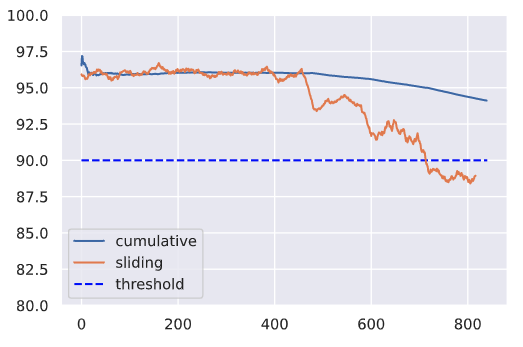## 延迟的结果

• 设计一个可以帮助估计模型性能的近似信号。例如，在标签预测任务中，可以使用作者赋予项目的实际标签作为中间标签，直到验证了来自注释管道的标签。
• 标记实时数据集的一小部分以估计性能。该子集应尽量代表实时数据中的各种分布。

## 重要性加权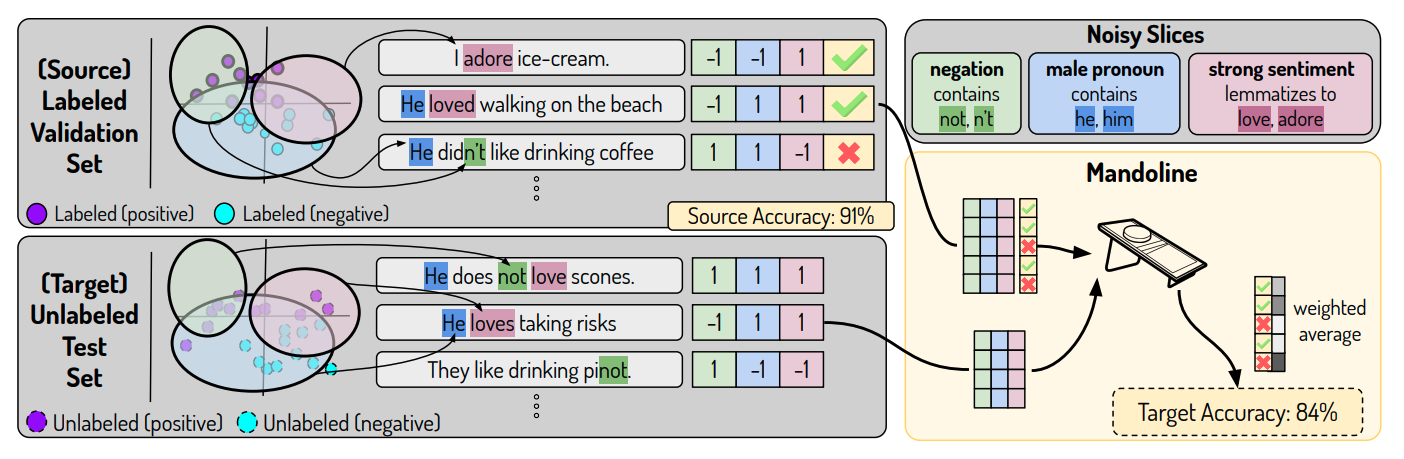Mandoline：分布转移下的模型评估

## 漂移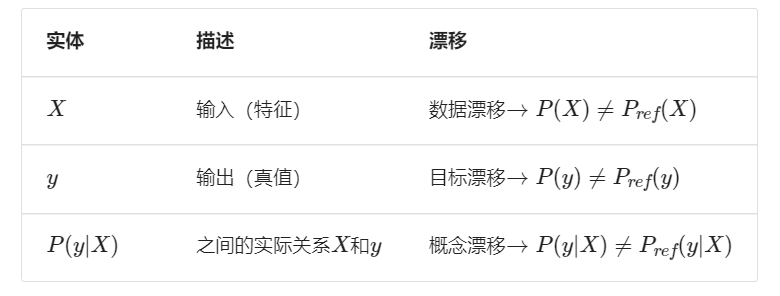### 数据漂移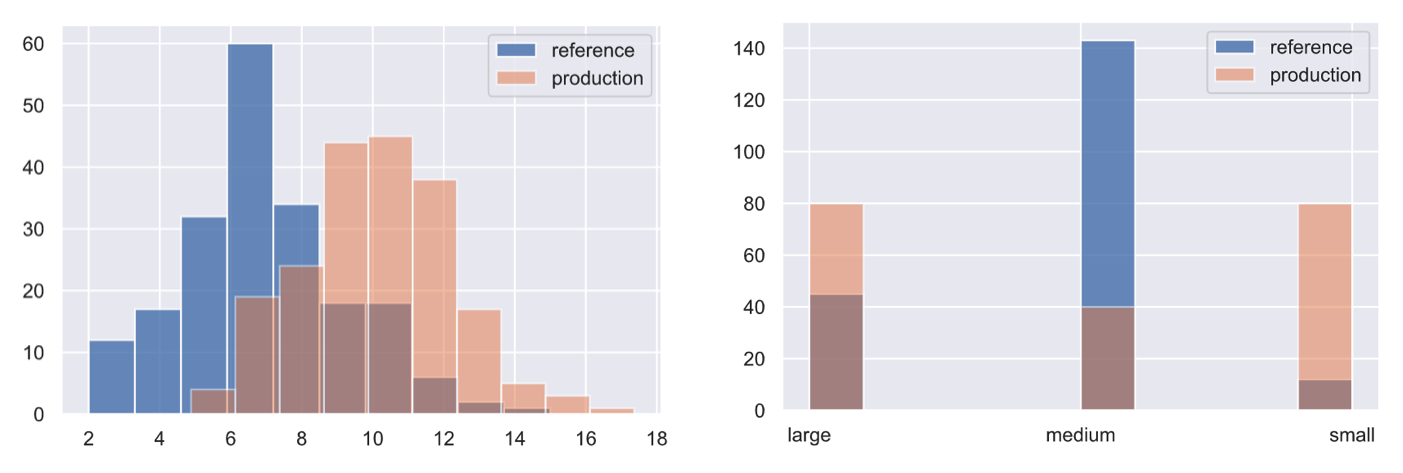### 概念漂移• 在一段时间内逐渐
• 由于外部事件而突然
• 由于反复发生的事件而定期

## 定位漂移

• 参考窗口：用于比较生产数据分布以识别漂移的一组点。
• 测试窗口：与参考窗口比较以确定是否发生漂移的点集。

Scikit-multiflow提供了一个工具包，用于直接在流数据上进行概念漂移检测技术。该软件包提供了窗口化、移动平均功能（包括动态预处理），甚至还提供了一些围绕概念的方法，例如逐渐概念漂移

## 测量漂移

import great_expectations as ge
import json
import pandas as pd
from urllib.request import urlopen

# Load labeled projects
df = ge.dataset.PandasDataset(pd.merge(projects, tags, on="id"))
df["text"] = df.title + " " + df.description
df.drop(["title", "description"], axis=1, inplace=True)


### 期望

# Simulated production data
prod_df = ge.dataset.PandasDataset([{"text": "hello"}, {"text": 0}, {"text": "world"}])

# Expectation suite
df.expect_column_values_to_not_be_null(column="text")
df.expect_column_values_to_be_of_type(column="text", type_="str")
expectation_suite = df.get_expectation_suite()

# Validate reference data
df.validate(expectation_suite=expectation_suite, only_return_failures=True)["statistics"]


{‘evaluated_expectations’: 2, ‘success_percent’: 100.0, ‘successful_expectations’: 2, ‘unsuccessful_expectations’: 0}

# Validate production data
prod_df.validate(expectation_suite=expectation_suite, only_return_failures=True)["statistics"]


{‘evaluated_expectations’: 2, ‘success_percent’: 50.0, ‘successful_expectations’: 1, ‘unsuccessful_expectations’: 1}

### 单变量

#### Kolmogorov-Smirnov (KS) 测试

KS 检验确定两个分布的累积密度函数之间的最大距离。在这里，将测量两个不同数据子集之间输入文本特征的大小是否存在任何偏差。

TIPS

from alibi_detect.cd import KSDrift
# Reference
df["num_tokens"] = df.text.apply(lambda x: len(x.split(" ")))
ref = df["num_tokens"][0:200].to_numpy()
plt.hist(ref, alpha=0.75, label="reference")
plt.legend()
plt.show()

# Initialize drift detector
length_drift_detector = KSDrift(ref, p_val=0.01)

# No drift
no_drift = df["num_tokens"][200:400].to_numpy()
plt.hist(ref, alpha=0.75, label="reference")
plt.hist(no_drift, alpha=0.5, label="test")
plt.legend()
plt.show()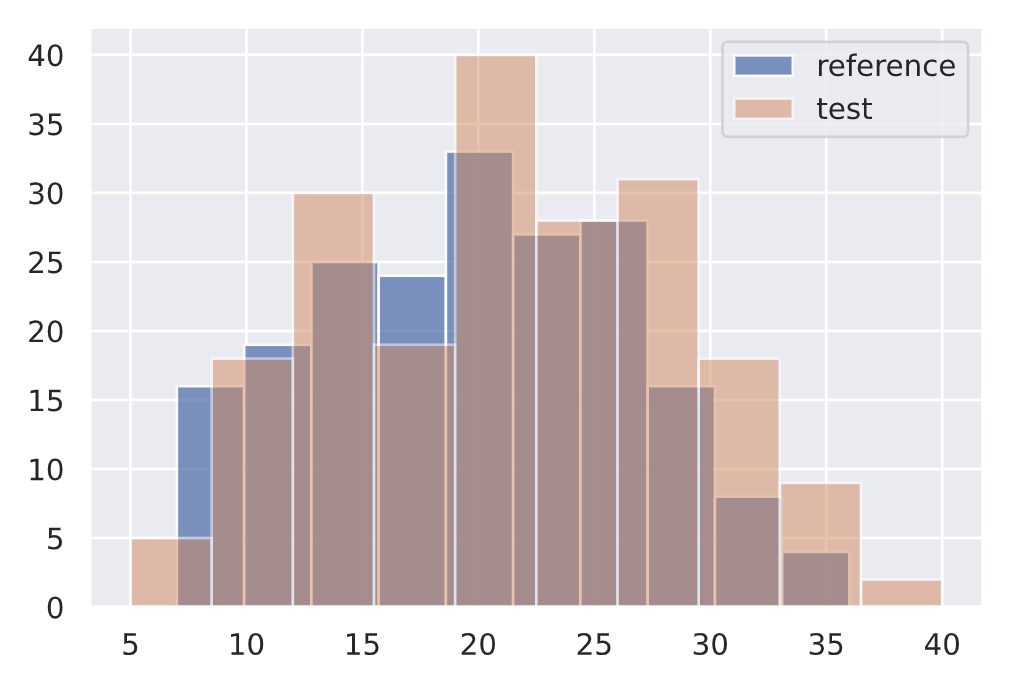length_drift_detector.predict(no_drift, return_p_val=True, return_distance=True)


{‘data’: {‘distance’: array([0.09], dtype=float32), ‘is_drift’: 0, ‘p_val’: array([0.3927307], dtype=float32), ‘threshold’: 0.01}, ‘meta’: {‘data_type’: None, ‘detector_type’: ‘offline’, ‘name’: ‘KSDrift’, ‘version’: ‘0.9.1’}}

↓ p 值 = ↑ 确信分布不同。

# Drift
drift = np.random.normal(30, 5, len(ref))
plt.hist(ref, alpha=0.75, label="reference")
plt.hist(drift, alpha=0.5, label="test")
plt.legend()
plt.show()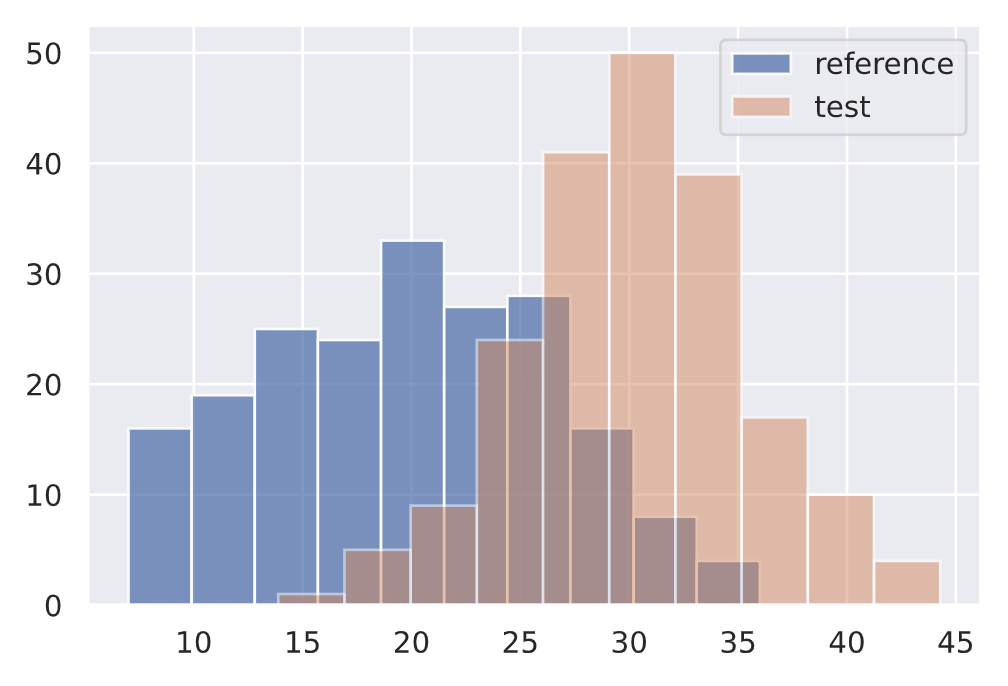length_drift_detector.predict(drift, return_p_val=True, return_distance=True)

{'data': {'distance': array([0.63], dtype=float32),
'is_drift': 1,
'p_val': array([6.7101775e-35], dtype=float32),
'threshold': 0.01},
'meta': {'data_type': None,
'detector_type': 'offline',
'name': 'KSDrift',
'version': '0.9.1'}}


#### 卡方检验

from alibi_detect.cd import ChiSquareDrift
# Reference
df.token_count = df.num_tokens.apply(lambda x: "small" if x <= 10 else ("medium" if x <=25 else "large"))
ref = df.token_count[0:200].to_numpy()
plt.hist(ref, alpha=0.75, label="reference")
plt.legend()

# Initialize drift detector
target_drift_detector = ChiSquareDrift(ref, p_val=0.01)

# No drift
no_drift = df.token_count[200:400].to_numpy()
plt.hist(ref, alpha=0.75, label="reference")
plt.hist(no_drift, alpha=0.5, label="test")
plt.legend()
plt.show()target_drift_detector.predict(no_drift, return_p_val=True, return_distance=True)


{‘data’: {‘distance’: array([4.135522], dtype=float32), ‘is_drift’: 0, ‘p_val’: array([0.12646863], dtype=float32), ‘threshold’: 0.01}, ‘meta’: {‘data_type’: None, ‘detector_type’: ‘offline’, ‘name’: ‘ChiSquareDrift’, ‘version’: ‘0.9.1’}}

# Drift
drift = np.array(["small"]*80 + ["medium"]*40 + ["large"]*80)
plt.hist(ref, alpha=0.75, label="reference")
plt.hist(drift, alpha=0.5, label="test")
plt.legend()
plt.show()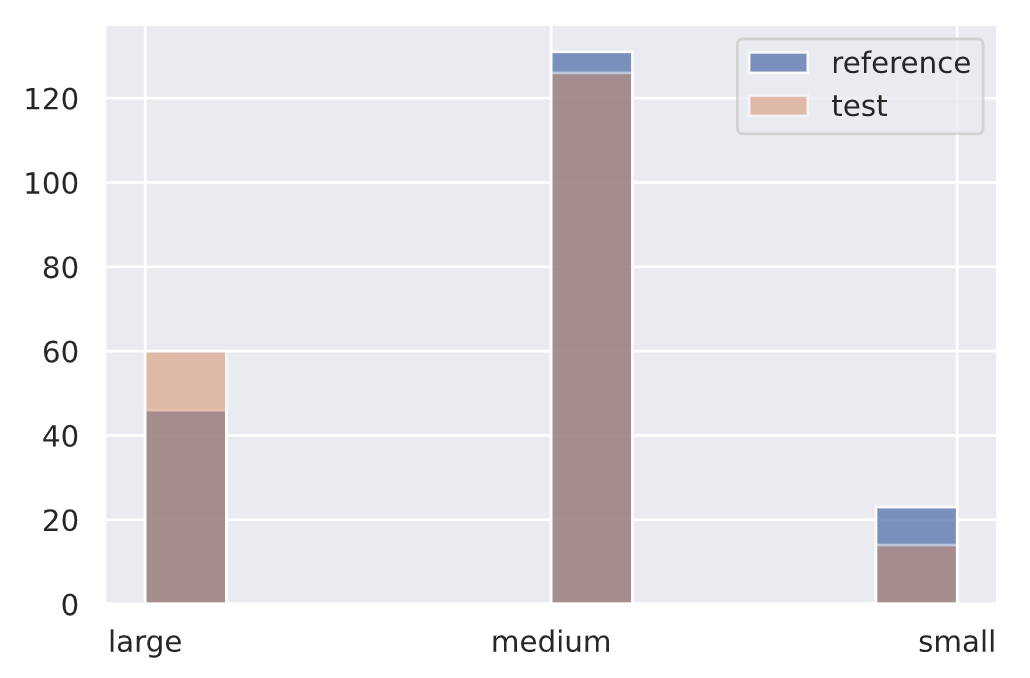target_drift_detector.predict(drift, return_p_val=True, return_distance=True)


{‘data’: {‘is_drift’: 1, ‘distance’: array([118.03355], dtype=float32), ‘p_val’: array([2.3406739e-26], dtype=float32), ‘threshold’: 0.01}, ‘meta’: {‘name’: ‘ChiSquareDrift’, ‘detector_type’: ‘offline’, ‘data_type’: None}}

### 多变量from transformers import AutoTokenizer
model_name = "allenai/scibert_scivocab_uncased"
tokenizer = AutoTokenizer.from_pretrained(model_name)
vocab_size = len(tokenizer)
print (vocab_size)


31090

# Tokenize inputs
ids = encoded_input["input_ids"]

# Decode
print (f"{ids}\n{tokenizer.decode(ids)}")


tensor([ 102, 2029, 467, 1778, 609, 137, 6446, 4857, 191, 1332, 2399、13572、19125、1983、147、1954、165、6240、205、185、 300、3717、7434、1262、121、537、201、137、1040、111、 545、121、4714、205、103、0、0、0、0、0、 0, 0, 0, 0, 0, 0, 0, 0, 0, 0, 0, 0, 0, 0, 0, 0, 0, 0, 0, 0, 0]) [CLS] comparison between yolo and rcnn on real world videos bringing theory to experiment is cool. we can easily train models in colab and find the results in minutes. [SEP] [PAD] [PAD] …

# Sub-word tokens
print (tokenizer.convert_ids_to_tokens(ids=ids))


[‘[CLS]’, ‘comparison’, ‘between’, ‘yo’, ‘##lo’, ‘and’, ‘rc’, ‘##nn’, ‘on’, ‘real’, ‘world’, ‘videos’, ‘bringing’, ‘theory’, ‘to’, ‘experiment’, ‘is’, ‘cool’, ‘.’, ‘we’, ‘can’, ‘easily’, ‘train’, ‘models’, ‘in’, ‘col’, ‘##ab’, ‘and’, ‘find’, ‘the’, ‘results’, ‘in’, ‘minutes’, ‘.’, ‘[SEP]’, ‘[PAD]’, ‘[PAD]’, …]

from alibi_detect.models.pytorch import TransformerEmbedding
# Embedding layer
emb_type = "hidden_state"
layers = [-x for x in range(1, 9)]  # last 8 layers
embedding_layer = TransformerEmbedding(model_name, emb_type, layers)

# Embedding dimension
embedding_dim = embedding_layer.model.embeddings.word_embeddings.embedding_dim
embedding_dim


768

#### 降维

• 主成分分析（PCA）：保持数据集可变性的正交变换。
• 自动编码器（AE）：消耗输入并尝试从较低维空间重建它同时最小化错误的网络。这些可以是经过训练的，也可以是未经训练的（大声失败的论文建议未经训练）。
• 黑盒移位检测器（BBSD）：在训练数据上训练的实际模型可以用作降维器。可以使用 softmax 输出（多变量）或实际预测（单变量）。
import torch
import torch.nn as nn
# Device
device = torch.device("cuda" if torch.cuda.is_available() else "cpu")
print(device)

# Untrained autoencoder (UAE) reducer
encoder_dim = 32
reducer = nn.Sequential(
embedding_layer,
nn.Linear(embedding_dim, 256),
nn.ReLU(),
nn.Linear(256, encoder_dim)
).to(device).eval()


from alibi_detect.cd.pytorch import preprocess_drift
from functools import partial
# Preprocessing with the reducer
max_len = 100
batch_size = 32
preprocess_fn = partial(preprocess_drift, model=reducer, tokenizer=tokenizer,
max_len=max_len, batch_size=batch_size, device=device)


#### 最大平均差异 (MMD)

from alibi_detect.cd import MMDDrift
# Initialize drift detector
mmd_drift_detector = MMDDrift(ref, backend="pytorch", p_val=.01, preprocess_fn=preprocess_fn)# No drift
no_drift = df.text[200:400].to_list()
mmd_drift_detector.predict(no_drift)


{‘data’: {‘distance’: 0.0021169185638427734, ‘distance_threshold’: 0.0032651424, ‘is_drift’: 0, ‘p_val’: 0.05999999865889549, ‘threshold’: 0.01}, ‘meta’: {‘backend’: ‘pytorch’, ‘data_type’: None, ‘detector_type’: ‘offline’, ‘name’: ‘MMDDriftTorch’, ‘version’: ‘0.9.1’}}

# Drift
drift = ["UNK " + text for text in no_drift]
mmd_drift_detector.predict(drift)


## 在线的

from alibi_detect.cd import MMDDriftOnline
# Online MMD drift detector
ref = df.text[0:800].to_list()
online_mmd_drift_detector = MMDDriftOnline(
ref, ert=400, window_size=200, backend="pytorch", preprocess_fn=preprocess_fn)


Generating permutations of kernel matrix.. 100%|██████████| 1000/1000 [00:00<00:00, 13784.22it/s] Computing thresholds: 100%|██████████| 200/200 [00:32<00:00, 6.11it/s]

def simulate_production(test_window):
i = 0
online_mmd_drift_detector.reset()
for text in test_window:
result = online_mmd_drift_detector.predict(text)
is_drift = result["data"]["is_drift"]
if is_drift:
break
else:
i += 1
print (f"{i} steps")

# Normal
test_window = df.text[800:]
simulate_production(test_window)


27 steps

# Drift
test_window = "UNK" * len(df.text[800:])
simulate_production(test_window)


11 steps

## 异常值from alibi_detect.od import OutlierVAE
X_train = (n_samples, n_features)
outlier_detector = OutlierVAE(
threshold=0.05,
encoder_net=encoder,
decoder_net=decoder,
latent_dim=512
)
outlier_detector.fit(X_train, epochs=50)
outlier_detector.infer_threshold(X, threshold_perc=95)  # infer from % outliers
preds = outlier_detector.predict(X, outlier_type="instance", outlier_perc=75)


## 解决方案

### 警报

• 固定值/范围，用于具体了解预期上限/下限的情况。

if percentage_unk_tokens > 5%:

• 预测阈值取决于先前的输入、时间等。

if current_f1 < forecast_f1(current_time):

• 不同漂移检测器的适当 p 值（↓ p 值 = ↑ 确信分布不同）。

from alibi_detect.cd import KSDrift
length_drift_detector = KSDrift(reference, p_val=0.01)


### 检查

• 触发的特定警报
• 相关元数据（时间、输入、输出等）
• 失败的阈值/期望
• 进行的漂移检测测试
• 来自参考和测试窗口的数据
• 相关时间窗口的日志记录
# Sample alerting ticket
{
"threshold": 0.05,
"measurement": "KSDrift",
"distance": 0.86,
"p_val": 0.03,
"reference": [],
"target": [],
"logs": ...
}


### 行为

• 确保所有数据预期均已通过。
• 确认没有数据架构更改。
• 在新的移位数据集上重新训练模型。
• 将参考窗口移动到更新的数据或赋予它更多的权重。
• 确定异常值是否是潜在的有效数据点。

## 生产## 参考@article{madewithml,
author       = {Goku Mohandas},
title        = { Made With ML },